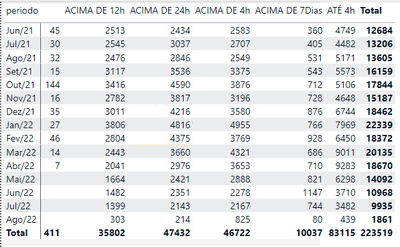cancel
Showing results for
Did you mean:Helper I

## achar o percentual entre total de FAIXA TEMPO por periodo dividido pelo valor total de cada período

Como calcular o valor da coluna (FAIXA TEMPO) distribuído em colunas abaixo com valor de cada periodo (ACIMA DE 24H, AC DE 4H ETC...) dividido pelo valor total de cada período?

O Objetivo é achar o percentual entre as FAIXA TEMPO dividido  pelo valor total de cada período

exemplo: Jun/21 --> Em branco (45/12684) Acima de 12h (2513/12684) Acima de 24h (2434/12684) Acima de 4h (2583/12684)

21/jul--> Em branco (30/13206) Acima de 12h (2545/13206) Acima de 24h (3037/13206) Acima de 4h (2707/13206)1 ACCEPTED SOLUTIONRegular Visitor

Hi @Victor1986 ,

Below is my test table:Create five measure and add it to table visual:

``````Total =
SUMX (
'Table 1',
[Em branco] + [DE 12h] + [DE 24h] + [DE 4h] + [DE 7Dias] + [ATE 4h]
)``````
``````Em/Total =
VAR cur_em =
SELECTEDVALUE ( 'Table 1'[Em branco] )
VAR a =
DIVIDE ( cur_em, 'Table'[Total] )
RETURN
a``````
``````DE 12h/Total =
VAR cur_12 =
SELECTEDVALUE ( 'Table 1'[DE 12h] )
VAR a =
DIVIDE ( cur_12, 'Table'[Total] )
RETURN
a``````
``````DE24/Total =
VAR cur_24 =
SELECTEDVALUE ( 'Table 1'[DE 24h] )
VAR a =
DIVIDE ( cur_24, 'Table'[Total] )
RETURN
a``````
``````DE 4h/Total =
VAR cur_4 =
SELECTEDVALUE ( 'Table 1'[DE 4h] )
VAR a =
DIVIDE ( cur_4, 'Table'[Total] )
RETURN
a``````If this post helps, then please consider Accept it as the solution to help the other members find it more quickly.

3 REPLIES 3Regular Visitor

Hi @Victor1986 ,

Below is my test table:Create five measure and add it to table visual:

``````Total =
SUMX (
'Table 1',
[Em branco] + [DE 12h] + [DE 24h] + [DE 4h] + [DE 7Dias] + [ATE 4h]
)``````
``````Em/Total =
VAR cur_em =
SELECTEDVALUE ( 'Table 1'[Em branco] )
VAR a =
DIVIDE ( cur_em, 'Table'[Total] )
RETURN
a``````
``````DE 12h/Total =
VAR cur_12 =
SELECTEDVALUE ( 'Table 1'[DE 12h] )
VAR a =
DIVIDE ( cur_12, 'Table'[Total] )
RETURN
a``````
``````DE24/Total =
VAR cur_24 =
SELECTEDVALUE ( 'Table 1'[DE 24h] )
VAR a =
DIVIDE ( cur_24, 'Table'[Total] )
RETURN
a``````
``````DE 4h/Total =
VAR cur_4 =
SELECTEDVALUE ( 'Table 1'[DE 4h] )
VAR a =
DIVIDE ( cur_4, 'Table'[Total] )
RETURN
a``````If this post helps, then please consider Accept it as the solution to help the other members find it more quickly.Helper I

@Luca_Yu como que voce montou a sua tabela de teste?Super User

Please paste the data into a table in your post or use one of the file services like OneDrive or Google Drive. I cannot use screenshots of your source data.
Please show the expected outcome based on the sample data you provided. Screenshots of the expected outcome are ok.Announcements#### Exclusive opportunity for Women!

Join us for a free, hands-on Microsoft workshop led by women trainers for women where you will learn how to build a Dashboard in a Day!#### Power Platform Conference-Power BI and Fabric Sessions

Join us Oct 1 - 6 in Las Vegas for the Microsoft Power Platform Conference.Top Solution Authors
Top Kudoed Authors
Users online (1,904)